Courses

# Extra Questions- Visualising Solid Shapes Class 8 Notes | EduRev

## Mathematics (Maths) Class 8

Created by: Full Circle

## Class 8 : Extra Questions- Visualising Solid Shapes Class 8 Notes | EduRev

The document Extra Questions- Visualising Solid Shapes Class 8 Notes | EduRev is a part of the Class 8 Course Mathematics (Maths) Class 8.
All you need of Class 8 at this link: Class 8

Question 1: Which of the following solids are not polyhedrons?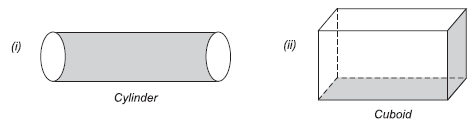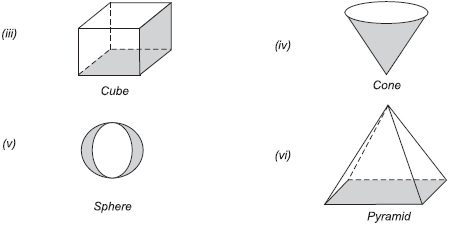Solution:
(i) A cylinder is not a polyhedron.
(ii) A cuboid is a polyhedron.
(iii) A cube is a polyhedron.
(iv) A cone is not a polyhedron.
(v) A sphere is not a polyhedron.
(vi) A pyramid is a polyhedron.

Question 2: Why the following solids are not polyhedron?
(i) A sphere.
(ii) A cone.
(iii) A cylinder.
Solution: Since, a polyhedron is a solid shape bounded by polygons. However,
(i) a sphere,
(ii) a cone and
(iii) a cylinder are not polyhedron because they are made of polygons, i.e. their faces are not polygons.

Question 3: Name the following polyhedron.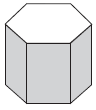How many faces, vertiecs and edges of this solid are there?
Solution: ∵ The ends (bases) of the given solid are congruent rectilinear figure each of six sides.
∴ It is a hexagonal prism.
In a hexagonal prism, we have:
The number of faces = 8
The number of edges = 18
The number of vertices = 12

Question 4: What Euler’s formula? Verify the Euler’s formula for a pentagonal prism.
Solution: If a polyhedron is having number of faces as F, number of edges as E and the number of vertices as V, then the relationship
F + V = E + 2
is known as Euler’s formula. Following figure is a solid pentagonal prism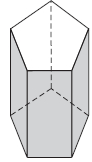It has:
Number of faces     (F) = 7
Number of edges    (E) = 15
Number of vertices (V) = 10
Substituting the values of F, E and V in the relation,
F + V = E + 2
we have
7 + 10 = 15 + 2
⇒                           17 = 17
Which is true, the Euler’s formula is verified.

Question 5: A polyhedron is having 8 vertices and 12 edges. How many faces of it are there?
Solution: Number of vertices (V) = 8
Number of edges (E) = 12
Let the number of faces = F
Now, using Euler’s formula
F + V = E + 2
we have
F + 8  = 12 + 2
⇒                                          F + 8 = 14
⇒                                                F = 14 – 8 ⇒ F = 6
Thus, the required number of faces = 6.

Question 6: An icosahedron is having 20 triangular faces and 12 vertices. Find the number of its edges.
Solution: Here:
Number of faces (F) = 20
Number of vertices (V) = 12
Let the number of edges be E.
∴ Using Euler’s formula, we have
F + V = E + 2
⇒                      20 + 12 = E + 2
⇒                              32 = E + 2
⇒                               E = 32 – 2 = 30
Thus, the required number of edges = 30.

Question 7: What is the least number of planes that can enclose a solid? Name the simplest regular polyhedron and verify Euler’s formula for it.
Solution: At least 4 planes can form to enclose a solid. Tetrahedron is the simple polyhedron. Following figure represents a simplest solid, called tetrahedron.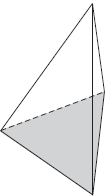A tetrahedron has:
4 triangular faces, i.e. F = 4
4 vertices,             i.e. V = 4
6 edges,                i.e. E = 6
Now, substituting the values of F, V and E in Euler’s formula, i.e.
F + V = E + 2
we have
4 + 4 = 6 + 2
⇒                         8 = 8, which is true.
Thus, Euler’s formula is verified for a tetrahedron.

,

,

,

,

,

,

,

,

,

,

,

,

,

,

,

,

,

,

,

,

,

;